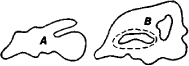# Multiply Connected Region

## multiply connected region

[′məl·tə·plē kə¦nek·təd ′rē·jən]
(mathematics)
An open set in the plane which has holes in it.
McGraw-Hill Dictionary of Scientific & Technical Terms, 6E, Copyright © 2003 by The McGraw-Hill Companies, Inc.
The following article is from The Great Soviet Encyclopedia (1979). It might be outdated or ideologically biased.

## Multiply Connected Region

in mathematics, a region in which there exist closed curves that cannot be contracted to a point within the region. In Figure 1, the region A is a simply connected region and the region B is a multiply connected region. A curve that cannot be contracted to a point within B is shown by the broken line.Figure 1

Mentioned in ?
References in periodicals archive ?
Nasser, "Annulus with circular slit map of bounded multiply connected regions via integral equation method," Bulletin of the Malaysian Mathematical Sciences Society.
--, Numerical conformal mapping of multiply connected regions onto the fifth category of Koebe's canonical slit regions, J.
Features include meshing of multiply connected regions with multiple levels of nested internal boundaries, external boundaries representing arbitrary combinations of line segments and arcs, internal points, and internal entities.
NASSER, Solving a mixed boundary value problem via an integral equation with adjoint generalized Neumann kernel in bounded multiply connected regions, in Proceedings of the 20th National Symposium on Mathematical Sciences, Malaysia, A.
NASSER, A boundary integral equation for conformal mapping of bounded multiply connected regions, Comput.
--, The Riemann-Hilbert problem and the generalized Neumann kernel on unbounded multiply connected regions, The University Researcher (IBB University Journal), 20 (2009), pp.
--, Numerical conformal mapping of multiply connected regions onto the second, third and fourth categories of Koebe's canonical slit domains, J.
AL-SHIHRI, A fast boundary integral equation method for conformal mapping of multiply connected regions, SIAM J.
ALEJAILY, Boundary integral equation with the generalized Neumann kernel for Laplace's equation in multiply connected regions, Appl.
NASSER, The Riemann-Hilbert problem and the generalized Neumann kernel on multiply connected regions, J.
NASSER, Numerical conformal mapping and its inverse of unbounded multiply connected regions onto logarithmic spiral slit regions and rectilinear slit regions, Proc.
Nasser, Parallel slits map of bounded multiply connected regions, J.

Site: Follow: Share:
Open / Close